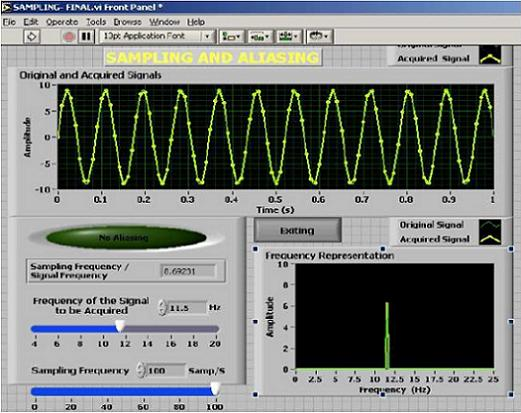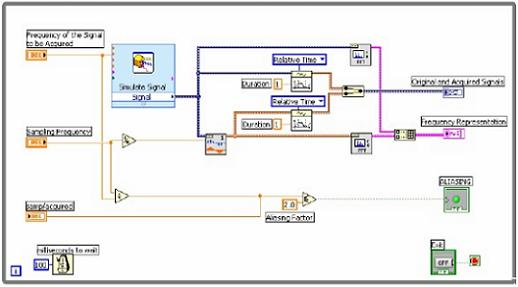# RF Simulation Demo: Sampling Theorem

Publish Date: Nov 05, 2006 | 15 Ratings | 3.93 out of 5 | Print | Submit your review

## Overview

The sampling theorem states that, if the sampling rate in any pulse modulation system exceeds twice the maximum signal frequency, the original signal can be reconstructed in the receiver with minimal distortion. The sampling theorem is used in practice to determine minimum sampling speeds.

### Procedure:

1. Place the Simulate Signal VI in the block diagram and configure it with signal type and frequency.

2. Place a numeric control for sampling frequency in front panel.

3. Place the arithmetic VI in the block diagram in order to calculate the aliasing effect.

4. Place the Resample Waveform VI in the block diagram to do sampling the simulated signal.

5. Place the FFT function VI in the block diagram in order to get the frequency waveform of the simulated signal.

6. Place the waveform graphs in the front panel and connect the blocks to output of each function in Block diagram.

7. Place the sub diagrams inside the while loop in order to repeat the operation until the stop button is enabled.### Observations:

1. Observe the sampling theorem concept by varying the simulated signal
frequency and sampling frequency

2. Observe the aliasing effect by varying the simulated frequency.

Attachments:

Sampling Theorem

Requirements

### Ratings

Rate this document